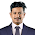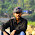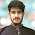Monday, December 21, 2009

Bitwise Operation: AND, OR, XOR, NOT

In computer, all the numbers and all the other data are stored using 2 based number system or in binary format. So, what we use to say a '5' in decimal (do we use decimal only because we have 10 figures? who knows...), a computer will represent it as '101', in fact, everything is represented as some sequence of 0s and 1s. We call this sequence a bit-string.

Bit-wise operations means working with the individual bits other than using the larger or default data types, like integers, floating points, characters, or some other complex types. C/C++ provides a programmer an efficient way to manipulate individual bits of a data with the help of commonly known logical operators like AND(&), OR(|), NOT(~), XOR(^), LEFT SHIFT(<<) and RIGHT SHIFT(>>) operators.

To be fluent with bit-wise operations, one needs to have a clear concepts about how data is stored in computer and what is a binary number system.

From a programming contest aspect, we'll explore bit-wise operations for only integer types (int in C/C++) and as this is almost 2010, we'll consider int as a 32 bit data type. But for the ease of writing, sometimes only the lowest 16 bits will be shown. The above operators works on bit-by-bit, i.e. during bit-wise operation, we have to align right the bits! (just like addition, multiplication,...) The shorter bits always have leading 0s at the front. And another important thing... we do not need to convert the integers to the binary form ourselves, when we use the operators, they will be automatically evaluated and the following examples shows the underlying activities... :P

& (AND) operator

This table will clearly explains the bit-wise operator & (AND):
`0 & 0 = 00 & 1 = 01 & 0 = 01 & 1 = 1`

Example:
What is the value of 24052 & 4978 ?
To solve this, we have to consider the operations in base 2.
So, we imagine both numbers in base 2 and align right the bits, as shown below using 16 bits. Now, AND the numbers : (shorter bits can have leading zeros at the front):
`101110111110100 ⇒ 24052001001101110010 ⇒ 4978--------------- &001000101110000 ⇒ 4464`

Each bit-column is AND-ed using the AND table above.

| (OR) operator

This table summarizes the OR operations :
`0 | 0 = 00 | 1 = 11 | 0 = 11 | 1 = 1`

Now, using the same example above, let's do it using | (OR) operator :
`101110111110100 ⇒ 24052001001101110010 ⇒ 4978--------------- |101111111110110 ⇒ 24566`

Each bit-column is OR-ed using the OR table above.

^ (XOR) operator

This table summarize the XOR operations :
`0 ^ 0 = 00 ^ 1 = 11 ^ 0 = 11 ^ 1 = 0`

Now, using the same example above, let's do using ^ (XOR) operator :
`101110111110100 ⇒ 24052001001101110010 ⇒ 4978--------------- ^100111010000110 ⇒ 20102`

Easy, isn't it?

~ (NOT) operator

This operator is different from the above operators. This operator is called unary operator, since it only needs one operand. The operators &, |, ^ are binary operators, since it takes 2 operands/number to be operated.

This ~ (NOT) operator, inverts all the bits in a variable. That is, changes all zeros into ones, and changes all ones into zeros.
Remember! that the result of this ~ (NOT) operation highly depends on the length of the bit-string.

Example:
`int a = 10;printf("%d\n", ~a);`

Surprisingly, the output is -11. But actually this is normal as most of the cases computer represents negative numbers in the 2's complement form. Look at the operation shown below using 16 bits:
`0000000000001010 ⇒ a(16 bits)---------------- ~1111111111110101 ⇒ -11`

This is correct! Because computer stores -11 as 1111111111110101 in binary! (in the 2's complement form). Even if we use 32 bits representation, it is still the 2's complement form of -11;
`-11(10) = 11111111111111111111111111110101(2)`

Anyway, if we do the same operation for unsigned int:
`unsigned int a = 10;printf("%u\n", ~a);`

Hah ha, don't be surprised if you get the output of the unsigned value of -11 is 4294967285.

If you use 32 bits, you actually will get -11 as 11111111111111111111111111110101 in signed binary representation:
`00000000000000000000000000001010 ⇒ 10 (32 bits)-------------------------------- ~11111111111111111111111111110101 ⇒ -11 (for signed) and -> 4294967285 (for unsigned)`

In the unsigned data type, all the bits are considered to be the part of the magnitude, there's no bit position reserved for sign bits.

Continue to Part 2

1.Thanks Vaia...

2.থ্যাংকস ভাই, unary and binary op সম্নধে ভালো ধারনা পাইলাম :D

3.4.